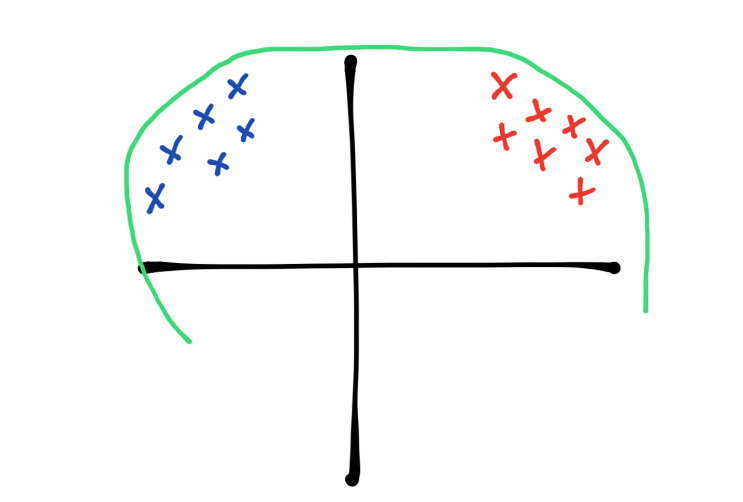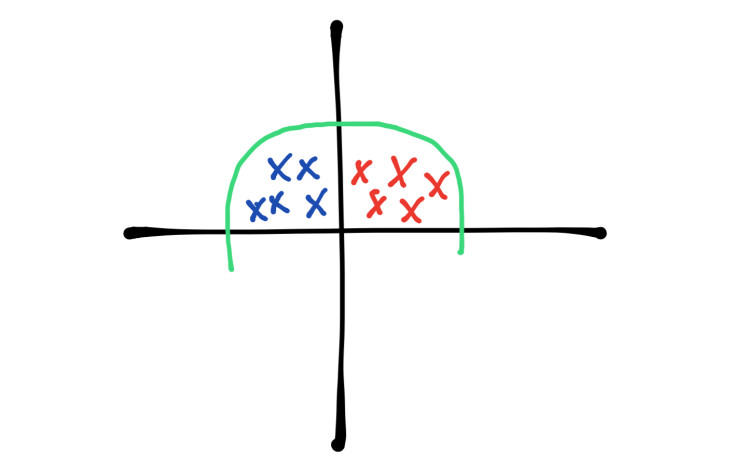April 24, 2019

Dropout and Batch Normalization

In this post I will explain two techniques that we can use to improve our neural networks, we usually use these two techniques together.

Dropout

Dropout deactivates a percentage of neurons of each layer randomly, each epoch the neurons will be randomly selected and deactivated, therefore the Forwardpropagation nor Backwardpropagation will not use these neurons to train the model. The purpose of this is avoid the neurons to create a strong relationship between each other and learn specific patrons that only work with the training dataset, if we deactivate randomly different neurons each epoch the model will have to find out good weights for all the neurons. If we use the model to predict classes in new data or to test it in the validation set we do not deactivate neurons, in Keras we can specify the percentage of deactivated neurons with a number between 0 and 1, we usually use 0.5 to indicate that we want the half of the neurons deactivated, in the following example we can see how to use Dropout in Keras:

model = Sequential()

We can notice that in the output layer we don’t have dropout due to the fact we need all the neurons activated in this layer. We can use dropout after each layer and we can also use it in the input layer .

If we are going to use dropout we should know two tricks:

• Since dropout deactivates a percentage of neurons we could need to add more layers to avoid Underfitting.
• We can use a bigger value for the learning rate hyperparameter.

Batch normalization

Batch normalization as its name suggest normalize each data batch, as we know we normalize the input data for example if we have images we change the range of values from 0-255 to 0-1 and this helps the neural network to obtain better results but we loss this normalization while the data goes through the model, with Batch normalization we can also normalize the data after each layer before passing the activation function.

Batch normalization also solves a problem called covariate shift, since we use batches to train a neural network we only pass a few amount of data each time, for example if we have images of some cars and these cars are blue and red, the batch should contain images of blue cars and red cars, we could achieve this merging all the images but this only helps the input layer.In the image above we can see the blue points as the blue cars and the red points as the red cars, both distributions are far from each other.If we normalize these images we can bring closer both distributions like in the image above, we can achieve this using batch normalization.

In Keras we can use the function BatchNormalization to implement batch normalization in our neural network:

model = Sequential()